International Journal of Mechanical Engineering and Applications
Volume 4, Issue 1, February 2016, Pages: 11-23

Research/Technical Note

Analysis and Design of a Scramjet Engine Inlet Operating from Mach 5 to Mach 10

Luu Hong Quan1, Nguyen Phu Hung2, Le Doan Quang3, Vu Ngoc Long1

1Department of Aeronautical and Space Engineering, School of Transportation Engineering, Hanoi University of Science and Technology, Hanoi, Vietnam

2The Ministry of Science and Technology – Hanoi University of Science and Technology, Hanoi, Vietnam

3Faculty of Aviation Technologies, Vietnam Aviation Academy, Ho Chi Minh City, Vietnam(L. H. Quan)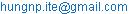(N. P. Hung)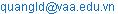(L. D. Quang)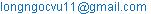(V. N. Long)

Luu Hong Quan, Nguyen Phu Hung, Le Doan Quang, Vu Ngoc Long. Analysis and Design of a Scramjet Engine Inlet Operating from Mach 5 to Mach 10. International Journal of Mechanical Engineering and Applications. Vol. 4, No. 1, 2016, pp. 11-23. doi: 10.11648/j.ijmea.20160401.12

Abstract: This paper gives a preliminary report of the analysis and design process of a scramjet engine inlet operating over a Mach number range from 5 to 10 without the use of variable geometry (moving parts) in order to find an optimal 2D geometry. An introduction of scramjet engine as well as its first component, the inlet, is given in the beginning and a number of basic inlet configurations are proposed. Inlet efficiency parameters and various design criteria are then explained, followed by a theoretical flow analysis utilizing some simplifying assumptions and the oblique shockwave relations. Next, 2D CFD simulations are carried out for some inlet geometries that are constructed based on the results of the theoretical analysis using the K-Omega SST turbulence model in Fluent to take into consideration boundary layer phenomena that the theoretical analysis is not able to cover. Lastly, a conclusion summarizing the design process is drawn and the optimal model is recommended.

Keywords: Scramjet, Hypersonic, Inlet, Oblique Shockwave, CFD

1. Introduction

Numerous programs aiming to develop aircrafts capable of hypersonic flight first appeared in the late 1950’s and early 1960’s and has developed unceasingly ever since . It has been found that rocket propelled vehicles were not a practical option for hypersonic flight due to the need of onboard oxidizer tank, resulting in low specific impulse. A more promising choice is an air breathing propulsion system and the best suitable air breathing engine cycle for hypersonic flight is scramjet – supersonic combustion ramjet, a variant of the ramjet engine cycle. Different from other types of air breathing engine like turbojet and turbofan, ramjet engine doesn’t rely on turbo machinery but shockwave for compression. As air passing through the engine inlet, it is compressed by shockwave and slowed down to a subsonic speed before entering the combustor where fuel is injected and burnt. Air is then accelerated through a nozzle to create thrust. Ramjet engine can only operate efficiently up to Mach 6 as at flight Mach number above 6, to achieve subsonic flow to the combustor, the compression ratio has to increase to a value at which shock losses become adversely substantial and the airflow temperature is so high that dissociation begins to occur in the nozzle, hence, less energy is extracted in form of thrust. Therefore, to sustain a usable amount of thrust at higher speed, the air entering the combustor has to be at lower pressure and temperature, meaning that it still moves at a supersonic speed. This modification to the ramjet engine is called supersonic combustion ramjet, or usually referred to by acronym as scramjet. Ground tests of scramjet engines have shown the potential to reach a maximum speed up to at least Mach 15 . The first scramjet development program was the NASA Hypersonic Research Engine (HRE) program which started in 1964, with about 52 tests completed . After this, many other programs in several countries such as Russia, France and Germany also began. Three prominent projects with successful flight test were the HyShot program by the University of Queensland in Australia that marked the first flight of a scramjet propelled aircraft in July 2002 , the Hyper-X program and the X-51 program. The aircraft designed in the Hyper-X program is called X-43. It has made two successful flight tests, the first one took place on March 2004 with the aircraft reaching a speed of Mach 7 and the second one on November 2004. In this second flight, X-43 got to nearly Mach 10, which set speed record . The most recent project involving scramjet is the SR-72, the successor of the SR-71 Blackbird. SR-72 is a hybrid turbojet – scramjet propelled aircraft which is expected to enter service by 2030 .

A scramjet engine consists of four major parts: inlet, isolator, combustor and nozzle. Throughout this paper, the scramjet engine is divided to different sections by the stations described in Fig. 1.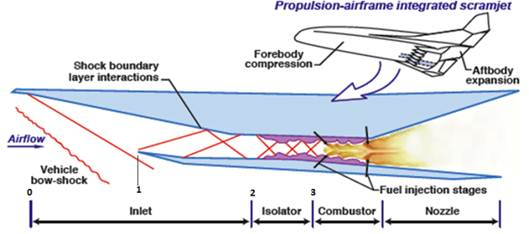Figure 1. Scramjet reference station designations.

The first component of a scramjet engine is the inlet. It is responsible for supplying a supersonic flow with suitable pressure, temperature and mass flow rate to the combustor for efficient combustion of fuel. Hence, the inlet is a critical component which affects greatly the overall efficiency of the whole scramjet engine. One major challenge of scramjet inlet design is the ability of the engine to operate over a wide range of Mach number without changing the geometry of the inlet. As the structure of the oblique shocks compressing the flow depends directly on the configuration of the inlet such as the number of ramps and the angles between each pair of adjacent ramps, the obvious solution for a scramjet engine to operate over a wide Mach number range is to change these parameters and thus change the geometry of the inlet. This however leads to a complex inlet structure that is an even bigger challenge. Therefore, the feasible solution is to keep a fixed inlet geometry that doesn’t degrade the performance of the inlet, thus the whole engine when the aircraft is not operating at the Mach number at which the inlet is designed. This study aims to tackle this challenge and attempts to design an inlet operating from Mach 5 to Mach 10 without the use of variable geometry.

In this study, the oblique shockwave relations serves as the theoretical base for inlet analysis. Various inlet design criteria can be found in tests and experiments carried out in past scramjet projects. Notably, powerful Solver tool of Excel and CFD turbulence modelling in Fluent allow us to optimize the performance of the inlet and to capture complex phenomena of the flow through the inlet.

2. Proposed Inlet Models

First, we need to determine the basic shape of the inlet. There are three types of scramjet inlet  as shown in Figs. 2-4.

Due to its shape, internal compression inlet generates a high amount of drag and it is not very easy to integrate the inlet with the whole vehicle. Moreover, the design of internal compression inlet is rather complicated because of complex flow field structure and the need for variable geometry to establish stable flow. In external compression inlet, in order for all oblique shock to be outside the inlet throat, flow has to enter the combustor at a large angle relative to the free stream flow. This causes the inlet cowl to be placed in the direction not parallel to the free stream, which results in high cowl drag. This downfall makes external compression inlet an unpopular choice in scramjet inlet design. Mixed compression inlet can resolve said problems, thus, our inlet will be designed based on this type.

The number of ramps is another important feature of an inlet as it determines the flow field structure through the inlet and will in turn affect the overall compression ratio and the efficiency of the inlet. Mixed compression inlet becomes even longer with more ramps. The growth in the size of the inlet leads to complex structure, high drag and thus, decreases the inlet performance. Various research in the past have considered inlet models with more than one ramps [1,5]. Consequently, calculation for a ramp number range from 2 to 6 will be done.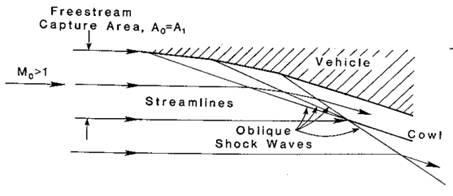Figure 2. External compression inlet.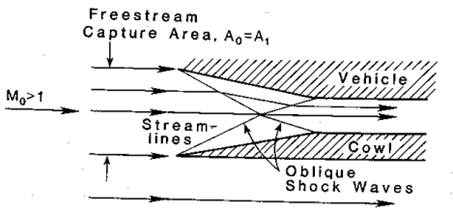Figure 3. External compression inlet.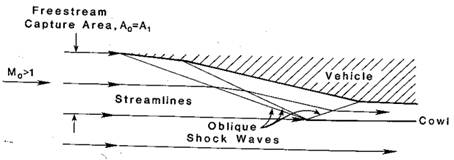Figure 4. External compression inlet.

3. Inlet Efficiency Parameters

Scramjet inlet performance can be assessed by various parameters . However, there are two that are most commonly used: total pressure ratio and kinetic energy efficiency. Total pressure ratio, also known as the total pressure recovery, is the ratio of the total pressure of the flow after the inlet (i.e. in front of the isolator) relative to that of the free stream :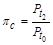(1)

Kinetic energy efficiency can be defined from the Molier diagram shown in Fig. 5. It is the ratio of the kinetic energy of the flow after the inlet if it expands isentropically to free stream pressure to the kinetic energy of the free stream :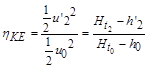(2)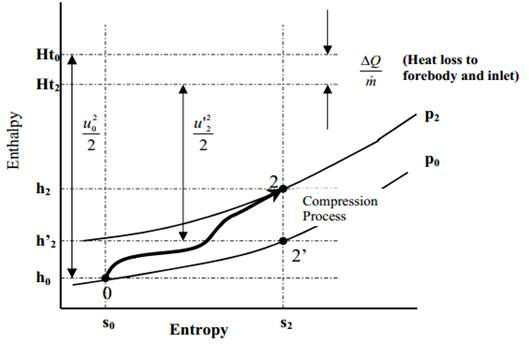Figure 5. Molier diagram of inlet compression process.

It can be seen from the diagram that air is compressed from point 0 to point 2 and if it expands isentropically to point 2’, we can derive the kinetic energy by subtracting the flow enthalpy from its total enthalpy. Note that when expanding isentropically from point 2, the flow loses an amount of energy due to heat loss, hence,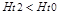. A variation of the kinetic energy efficiency which doesn’t take into account the heat loss is called adiabatic kinetic energy efficiency :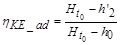(3)

According to ,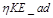can be calculated as follow: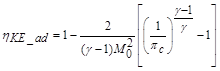(4)

4. Design Criteria

4.1. Requirement for a Robust Combustion Process

The combustion process takes time to finish. On the other hand, flow through the combustor moves at a high supersonic speed. Therefore, a specific length is required for a complete combustion process, which will in turn determine the length of the combustor. Short combustor is desired as the increase of the combustor length leads to the fact that more drag and heat load is placed upon the aircraft structure, decreasing dramatically the overall performance of the aircraft. To achieve this goal, the flow to the combustor need to reach certain value of pressure and temperature.

Combustion modelling is divided into an initial time for ignition and a further time for the combustion reaction to complete , which correspond to ignition length and reaction length respectively. Ignition length depends mainly on temperature and reaction length depends strongly on both temperature and pressure. An example of the correlation of ignition length and reaction length with temperature and pressure at a combustor velocity of 2400m/s in  is shown in Fig. 6.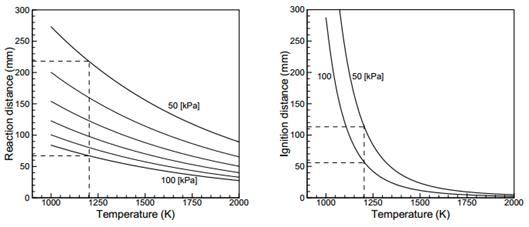Figure 6. Ignition length and reaction length of hydrogen at a combustors velocity of 2400m/s.

For hydrogen/air combustion, it has been found that short ignition length can be achieved with temperature well above 1000K, whereas the combustion reaction requires a pressure high enough (typically above 50kPa) to complete within an acceptable length. These constraints form a flow condition with pressure above 50kPa and temperature above 1000K in front of the combustor. However, local igniter system can provide air flow with a temperature high enough for a robust ignition process before it enters the combustor. Therefore, regarding the inlet design process in this paper, a minimum static pressure of 50kPa at the combustor entrance is needed but there is no minimum requirement for temperature.

The isolator between the inlet and the combustor can produce any pressure ranging from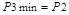to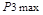that equals to the pressure after a normal shock behind the inlet . Thus, the pressure at the end of the inlet must higher than the value which, for a specific throat Mach number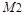, can produce a pressure of 50kPa after a normal shockwave so that it is possible to design an isolator that can provide a pressure high enough for a robust combustion process. For scramjet engine in particular, another constraint should be met: the Mach number after the isolator must greater than 1 so that the engine operates in supersonic combustion mode (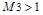). According to ,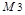can be calculated as follows: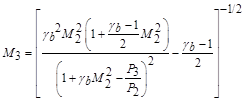(5)

Knowing the pressure and the Mach number after the inlet, we can determine the corresponding Mach number to a back pressure of the isolator that equals to 50kPa.

4.2. Dissociation in Front of the Nozzle

As air is slowed down through the inlet, its temperature increases considerably relative to the free stream temperature. The flow temperature is further augmented after air is burned with fuel in the combustor, and may reach the value at which dissociation become important in front of the nozzle. If temperature become too high, some portion of oxygen and nitrogen in the air may be dissociated and some portion of the combustion product remains unformed. In the rapid expansion process through the nozzle, the atoms in the air doesn’t have enough time to recombine utterly, thus, a portion of the energy received from the combustion process is not converted into thrust, which decreases the scramjet engine efficiency .

It is recommended in  that the maximum combustor entrance temperature should be in the range of 1440 – 1670K to prevent adverse effect of dissociation.

4.3. Self – Starting Inlet

A normally operating scramjet engine requires its inlet to operate in a started mode. The term "started" refers to the operating condition where flow phenomena in the internal portion of the inlet do not alter the capture characteristics of the inlet . Generally, unstarted inlet is caused by over – compressing the flow.

Results of extensive studies and testing on inlet starting have shown that internal contraction ratio and contraction ratio of the inlet all contributes to starting characteristics of a scramjet inlet. Internal contraction ratio is the ratio of the captured flow area at the plane of cowl closure to the throat area. Contraction ratio is the ratio of the captured flow area at the tip of the inlet and the throat area. It has been found that inlets will always be in started mode if its internal contraction ratio is lower than the Kantrowitz limit, which is determined by assuming that the flow is one – dimensional, isentropic and there is a normal shock at the plane of cowl closure and calculating the internal contraction ratio that will produce sonic flow at the throat . For an inlet whose internal contraction ratio is higher than the Kantrowitz limit, various experiments have shown that it can be in either started or unstarted mode . In contrast, inlets will always unstart if its inverted contraction ratio is lower than the value calculated from the following empirical formula for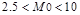: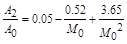(6)

The lower free stream Mach number, the higher this ratio is. Therefore, the geometry of our inlet will have an inverted contraction ratio of 0.1 because this value is higher than 0.092, which corresponds to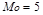, the lower end of the operating Mach number range.

4.4. Wide Operating Mach Number Range

One of the biggest challenge in scramjet inlet design is the capability of operating over a wide Mach number range without the use of variable geometry (rotating door, moving cowl…). With fixed geometry inlet, i.e. turning angles of the flow after each inlet ramp are constant, the shock angle (i.e. the angle formed by the flow direction and the oblique shockwave) decreases when the free stream Mach number is higher, thus changes the flow field structure along the inlet.

When the scramjet engine is operating in the on – design mode, all the external oblique shockwaves should focus at the cowl tip. The free stream Mach number corresponding to this operating condition is called the design Mach number.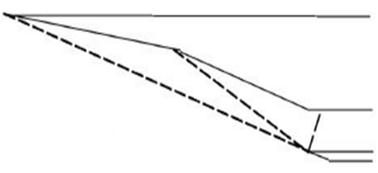Figure 7. Oblique shockwaves of scramjet inlet in on-design mode.

When the scramjet engine is operating at a flight Mach number below the design Mach number, flow spillage will happen.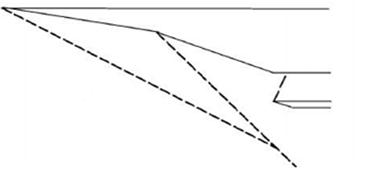Figure 8. Oblique shockwaves of scramjet inlet operating at flight Mach number lower than the design Mach number.

When the flight Mach number is above the design Mach number, the oblique shocks deviate into the inlet throat.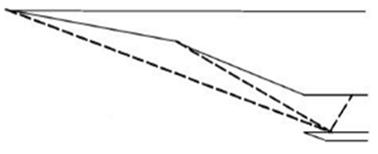Figure 9. Oblique shockwaves of scramjet inlet operating at flight Mach number higher than the design Mach number.

In this operating condition, shock – boundary layer interaction induces flow detachment and reattachment zones and high temperature spots inside the inlet throat, placing tremendous heat loads on the inlet structure. This operating condition is to be avoided due to the risk of flow instability and material failure.

The last two scenarios are two cases of off – design condition, which has to be taken into account in the inlet design process together with the on-design one. To stay away from the adverse operating condition, the design Mach number is chosen to be the highest one in the operating range, which is 10. For each inlet model proposed in this paper, simulation will be carried out for both this on – design Mach number and the lowest off – design one, which is 5.

4.5. Overall Scramjet Engine Efficiency

The relation of inlet compression characteristics and overall engine efficiency is achieved through engine cycle calculations, which involves not only the inlet but also the other components of the scramjet engine. The result of engine cycle calculation in  shows the correlation of overall efficiency with temperature ratio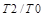at different free stream Mach numbers: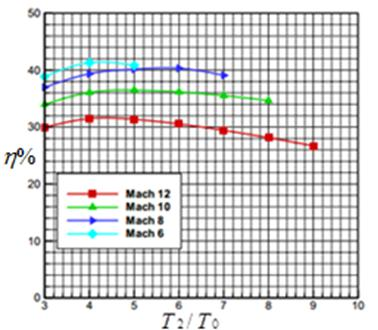Figure 10. Overall efficiency versus temperature ratio.

It can be inferred from the plot that overall efficiency decreases with flight Mach number and can drop to only 32% at Mach 10. For this reason, the temperature ratio across the inlet should be 5 when the engine is operating at Mach 10 in order to achieve the highest overall efficiency.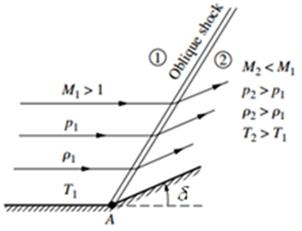Figure 11. Oblique shockwave.

5. One – Dimensional Flow Analysis

5.1. Oblique Shockwave Relations

The objective of the oblique shockwave relations is to find the shock angle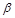, flow properties downstream of the shockwave based on known upstream condition and the turning angle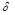, assuming that the flow through the inlet is one – dimensional, inviscid; air is perfect gas with constant gas index: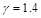and heat transfer between the flow and the inlet wall is neglected .

First,can be found using Eqn. (7) :(7)

Two values ofcan be found from Eqn. (7), one low and one higher which correspond to a weak and a strong oblique shock respectively . The lower one will be selected as strong shock leads to low total pressure ratio and thus low inlet efficiency.

With known free stream condition, flow properties after the oblique shock can be calculated using these following equations :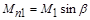(8)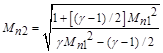(9)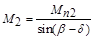(10)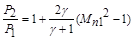(11)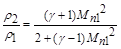(12)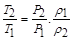(13)

5.2. Free Stream Condition

The aircraft is assumed to operate at a dynamic pressure of 50kPa, a value suitable for scramjet operation [1,5]. Mach number, static pressure, static temperature and density of the incoming air flow can be found based on the assumed flight dynamic pressure for each Mach number in the range from 5 to 10.

Table 1. Free stream condition.

 [kPa] [K] [kg/m3] 5 2.8602 220.7 0.045141 6 1.9868 224.8 0.030775 7 1.4598 229.3 0.022165 8 1.1179 233.3 0.016683 9 0.8833 236.9 0.012983 10 0.7143 240.2 0.010355

5.3. Calculation Process

Calculation is done in Excel using the oblique shock relations. The above free stream conditions serves as input parameters and the unknown parameters are the turning angles. The calculation process is similar for inlets with different ramp number.

For an inlet with (n-1) ramps, there are n oblique shocks, thus, there are n unknown parameters: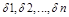(n = 3 – 7). The calculation process can be divided into 4 steps as follows:

Step 1: Give initial values for. Note that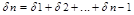according to the inlet geometry.

Step 2: Using the oblique shock relations and the free stream condition at Mach 5, calculate the properties of the flow after the first shock. Then, use the results to determine flow properties after the second shock using the same set of equations and the same process continues until the last shock. Properties of the flow from the free stream to the inlet throat are therefore determined and the static pressure ratio, the temperature ratio, the total pressure ratio and the adiabatic kinetic energy efficiency of the inlet can be calculated. Repeat this step for each flight Mach number from 6 to 10.

Step 3: In the Solver tool, set the objective to be maximum total pressure ratio at Mach 10 and set the constraints as follows:and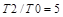(as discussed in section 4.5).

Step 4: Run the Solver tool to find. These are the values corresponding to the maximum total pressure ratio of the inlet and the highest overall scramjet engine efficiency at Mach 10. Note that the other parameters have also changed from the initial values that we calculated.

5.4. Theoretical Results and Discussions

Static pressure ratio, static temperature ratio, total pressure ratio and adiabatic kinetic energy efficiency are plotted versus flight Mach number for all five cases of ramp number in Figs. 12-15: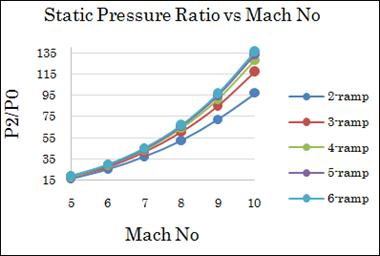Figure 12. Static pressure ratio versus flight Mach number.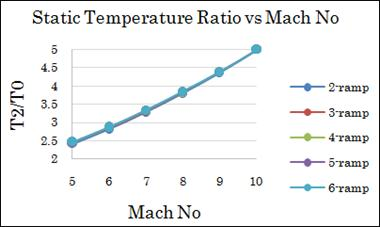Figure 13. Static temperature ratio versus flight Mach number.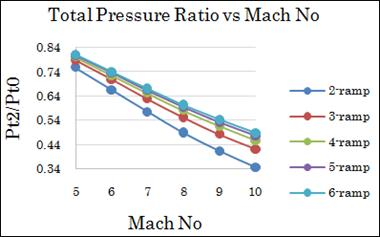Figure 14. Total pressure ratio versus flight Mach number.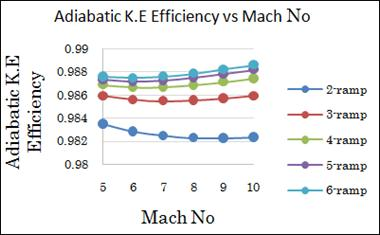Figure 15. Adiabatic kinetic energy efficiency versus flight Mach number.

The results regarding the static pressure ratio and static temperature ratio meet the said criteria as:

Static pressure ratio is high enough to produce supersonic flow with a pressure of 50kPa.

Maximum static temperature after the inlet is 1201K (at Mach 10), which is lower than the limit where dissociation starts to happen behind the combustor.

Up to this point of the design process, we can say that as the number of ramps increases, the inlet performance increases for the reason that:

Higher static pressure ratio means higher static pressure at the end of the inlet. This will provide better condition for combustion as discussed above.

There is a slight increase inat lower Mach number. This means higher overall scramjet engine efficiency according to Fig. 10.

Higher total pressure ratio and higher adiabatic kinetic energy efficiency.

However, the increasing rate of the inlet performance slows down substantially as the ramp number increases and becomes insignificant starting from the four – ramp case. Thus, the cases of five and six ramps are eliminated from the designing process due to the fact that the increase in inlet performance is inconsiderable compared to the increase in the size of the inlet (provided that the throat height is constant). Moreover, flow distortion after the inlet also increases with ramp number and the inlet may fail to meet one or more criteria described above, this will be demonstrated in the CFD results.

6. CFD Simulation

6.1. Inlet Geometries

Based on the angle of each oblique shock, the turning angle of each ramp of the inlet and assuming that the inlet throat height is equal to 25mm and the contraction ratio is 10, we can construct a 2D inlet geometry for each case of ramp number as shown in Figs. 16-18.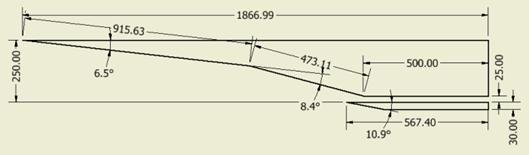Figure 16. 2 – ramp inlet geometry.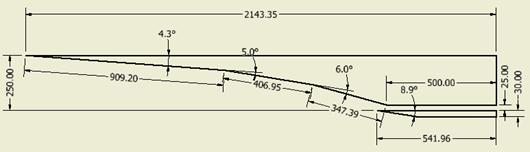Figure 17. 3 – ramp inlet geometry.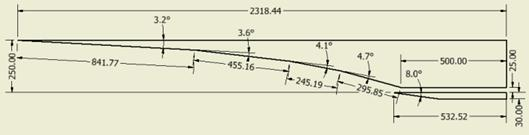Figure 18. 4 – ramp inlet geometry.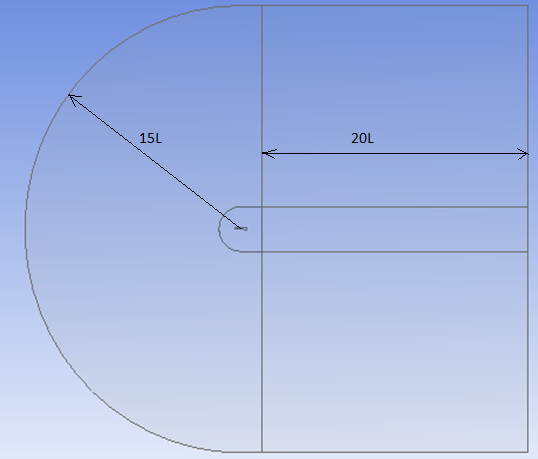Figure 19. Computational fluid domain.

6.2. Computational Domain and Mesh

The fluid domain is bounded by a semi – circle whose radius is about 15 times the inlet length and a rectangle downstream approximately 20 times longer than the inlet length. This fluid domain is far enough away from the inlet model to apply the pressure far field boundary condition in Fluent. The fluid domain is also divided into two fluid bodies: one inner body which will be meshed with finer unstructured quad elements to capture the inlet geometry, one outer body which will have structured mesh with quad elements growing larger as they are farther away from the inlet geometry. In addition, a boundary mesh of 15 layers will be generated around the inlet wall to better capture the boundary layer phenomenon. More than 100,000 elements are generated to achieve mesh-independent results.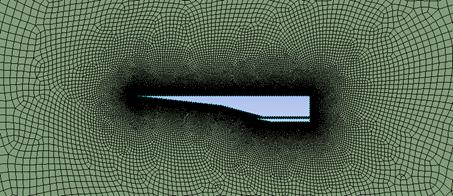Figure 20. 2 – ramp inlet mesh.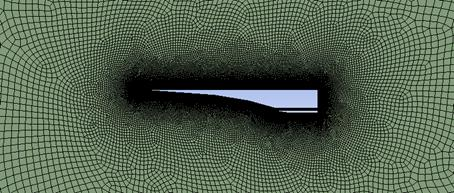Figure 21. 3 – ramp inlet mesh.Figure 22. 4 – ramp inlet mesh.

6.3. Boundary Condition

Two boundary conditions are created for the CFD simulation. The pressure far field boundary condition defines the Mach number and static pressure and static temperature of the free stream, these values are taken from Table. 1. The wall boundary condition is applied for the inlet wall.

6.4. CFD Results and Discussions

Numerical simulations at Mach 5 and Mach 10 are carried out using Fluent with the K – Omega SST turbulence model. Viscosity of the flow as well as shock – boundary layer interaction phenomenon are taken into account. These results will provide us with final data to determine the optimal inlet geometry.

2 – ramp inlet at Mach 5: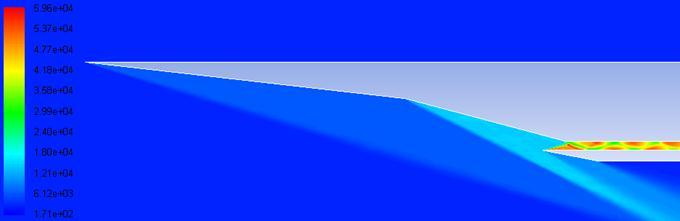Figure 23. Pressure contour.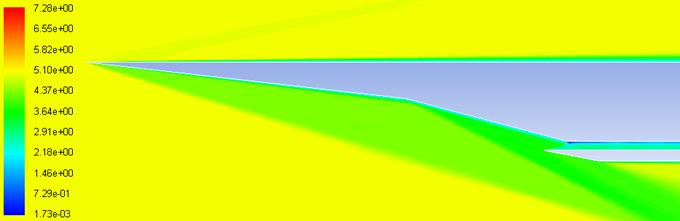Figure 24. Mach number contour.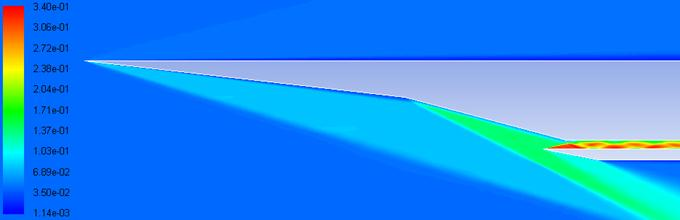Figure 25. Density contour.

3 – ramp inlet at Mach 5: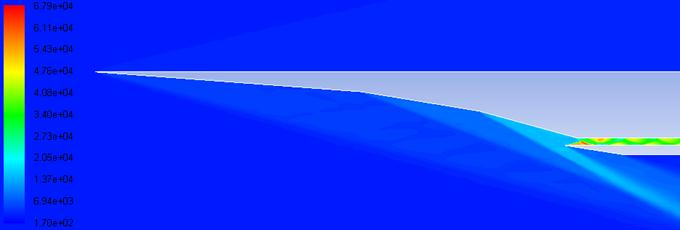Figure 26. Pressure contour.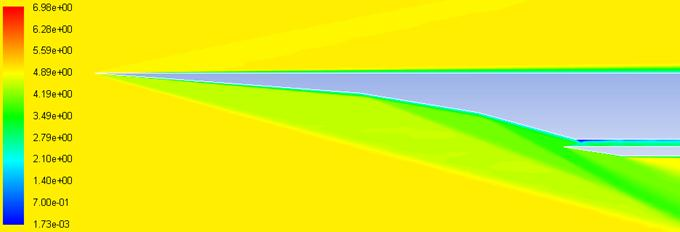Figure 27. Mach number contour.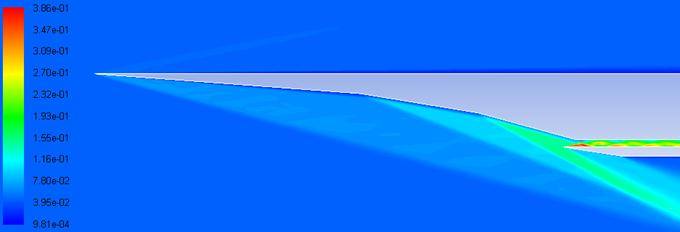Figure 28. Density contour.

The 4 – ramp case shows that the inlet is not self – starting. Thus, it is eliminated from the design process and only two cases are left for consideration.

2 – ramp inlet at Mach 10: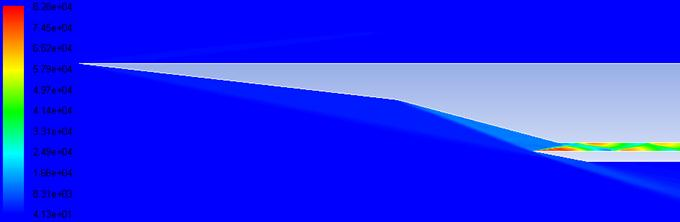Figure 29. Pressure contour.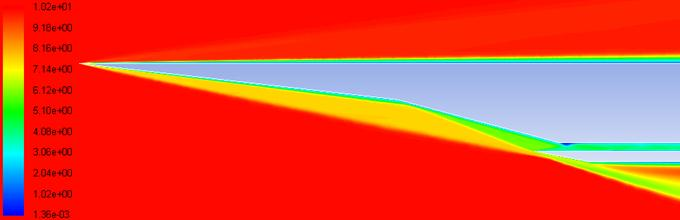Figure 30. Mach number contour.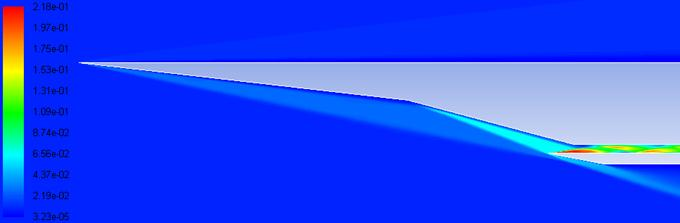Figure 31. Density contour.

3 – ramp inlet at Mach 10: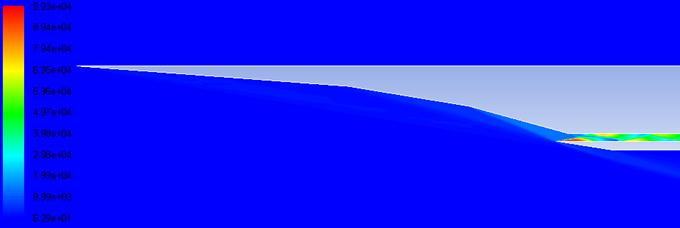Figure 32. Pressure contour.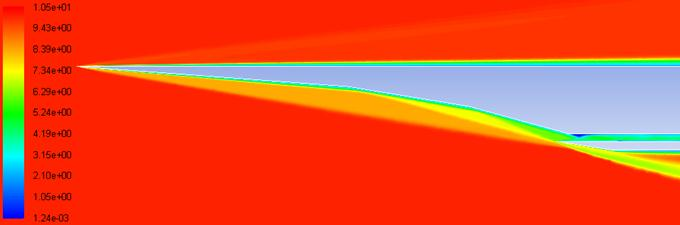Figure 33. Mach number contour.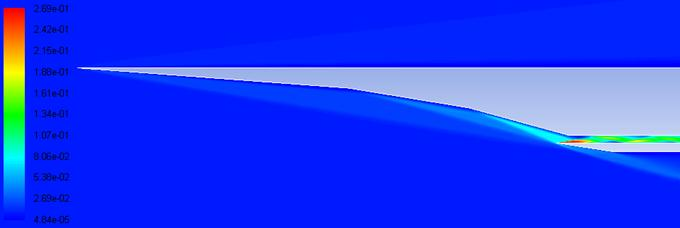Figure 34. Density contour.

The CFD results show minor difference from the theoretical analysis results. Below in Figs. 35-38 are pressure changes along the 2 – ramp inlet and the 3 – ramp inlet according to the theoretical results and the CFD results, the blue horizontal lines represent the pressure before and after each oblique shock as calculated by the oblique shockwave relations and the red curve signifies the pressure change along the inlet according to the CFD results: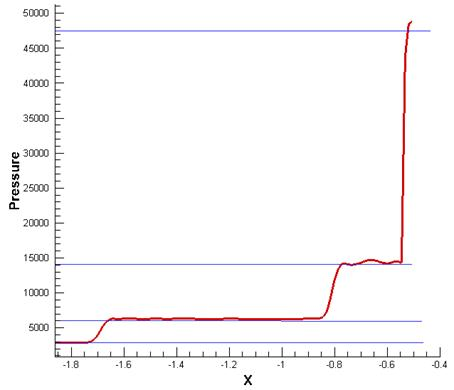Figure 35. CFD results and theoretical results comparison of 2 – ramp inlet at Mach 5.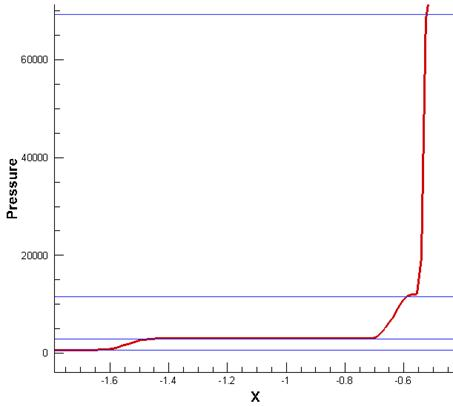Figure 36. CFD results and theoretical results comparison of 2 – ramp inlet at Mach 10.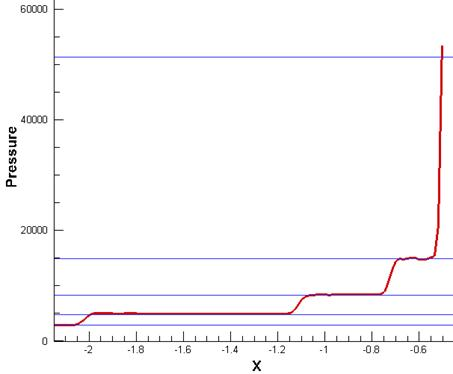Figure 37. CFD results and theoretical results comparison of 3 – ramp inlet at Mach 5.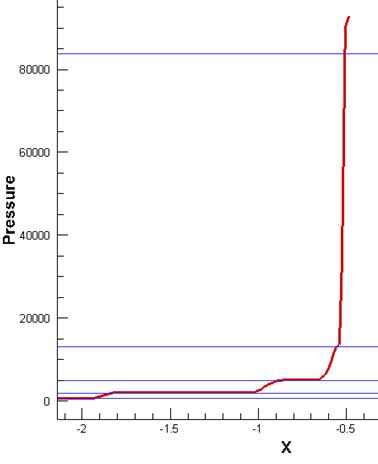Figure 38. CFD results and theoretical results comparison of 3 – ramp inlet at Mach 10.

These small differences are due to the thickness of the viscous boundary layer. This is, however, desirable as at on – design Mach number, the external shocks will be shifted away from the cowl lip by a small distance, avoiding a hot spot on the cowl tip.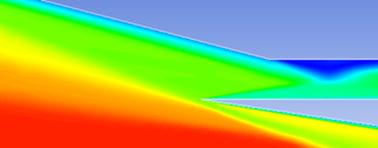Figure 39. Shockwaves deviate away from the cowl lip.

Another interesting phenomenon that can be observed in the CFD results is the shock – boundary layer interaction. Due to the limit of contraction ratio, the inlet throat height must be raised. As a result, the internal oblique shock hit the upper side of the inlet throat at a point behind the expansion fan. The pressure in the region in front of this point is low due to the expansion wave and the pressure in the region behind this point is high due to the internal oblique shock. This creates a middle region with strong adverse pressure gradient which induces flow separation. The deeper inside the inlet throat the internal shock is, the larger this middle region will be and the separation bubble will in turn become bigger, creating a further contraction after the inlet and may cause it to unstart. This is what happened with the 4 – ramp inlet.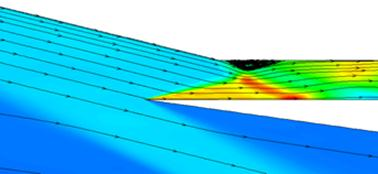Figure 40. Flow separation inside the inlet throat.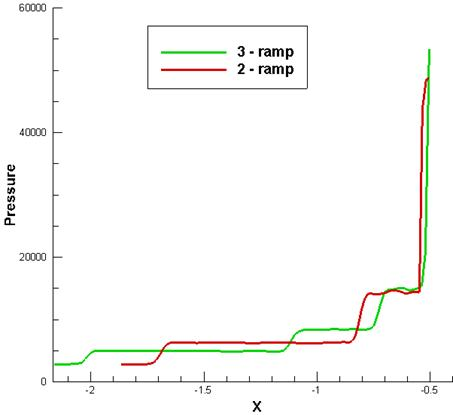Figure 41. Pressure changes of 2 – ramp inlet and 3 – ramp inlet at Mach 5.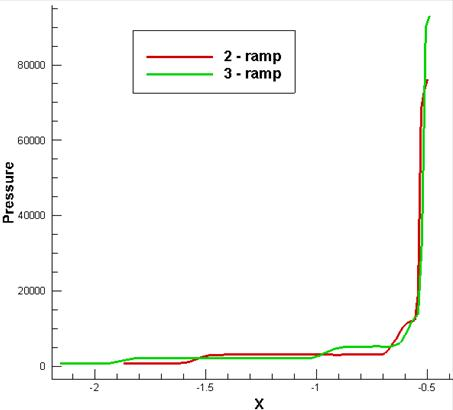Figure 42. Pressure changes of 2 – ramp inlet and 3 – ramp inlet at Mach 10.

There are two options to get the 4 – ramp inlet into started mode. The first one is to decrease the compression degree created by the inlet at, which will lead to altering the optimal geometry and thus will decrease the inlet efficiency. The second one is to decrease the contraction ratio by raising the inlet throat height. However, this also leads to an adverse consequence as the internal oblique shock will penetrate deeper into the inlet throat and create a bigger separation bubble that increases the flow non-uniformity after the inlet. Consequently, the 4 – ramp case is eliminated and only two cases are left for consideration.

Regarding the performance of 2 – ramp inlet and 3 – ramp inlet, the CFD results confirm the earlier statement made after the theoretical analysis, which implies that the 3 – ramp inlet is the optimal model for a scramjet inlet operating from Mach 5 to Mach 10. The graphs in Figs. 41-42 shows that 3 – ramp inlet can generate higher pressure than 2 – ramp inlet both at Mach 5 and Mach 10.

7. Conclusion

This paper has described the process of designing a scramjet inlet operating from Mach 5 to Mach 10 with no moving part, based on whichan inlet geometry with 3 ramps has been recommended as the optimal model. This is achieved through theoretical analysis using the oblique shockwave relations to find shock angle and turning angle which serve as data for geometry construction and to plot out curves of key performance parameters. Five inlet models with the number of ramps from 2 to 6 were subjects of this analysis and the results have narrowed down the options to three inlet models with 2, 3 and 4 ramps. CFD simulation using Fluent with the K – Omega SST turbulence model has shown that the 4 – ramp model fails to start at Mach 5 and given consistent results with the theoretical analysis for the other 2 cases. It has also confirmed that the performance of the 3 – ramp model is better than that of the 2 – ramp model.

Nomenclature

P = Pressure [kPa]

T = Temperature [K]

M = Mach number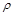= Density [kg/m3]

Pt = Total pressure [kPa]

u = Velocity [m/s]

H = Total enthalpy [J/kg]

h = Enthalpy [J/kg]

A = Area [m2]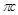= Total pressure ratio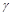= Gas index= Shock angle [degree]= Turning angle [degree]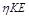= Kinetic energy efficiency= Adiabatic kinetic energy efficiency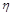= Overall efficiency

References

1. Michael K.Smart, Scramjet inlets, Centre for Hypersonics, The University of Queensland Brisbane 4072, Australia, September 2010.
2. Marta Marimon Mateu, Study of an Air – Breathing Engine for Hypersonic Flight, Polytechnic University of Catalonia, Spain, September 2013.
3. Elvia Thompson, Keith Henry and Leslie Williams, Faster Than a Speeding Bullet: Guinness Recognizes NASA Scramjet, NASA, June 2005.
4. Guy Norris, Skunk Works Reveals SR-71 Successor Plan, Aviation Week & Space Technology, November 2013.
5. Van Wei, D.M., edited by E.T.Curran, Scramjet Propulsion, Progress in Astronautics and Aeronautics, Vol 189, AIAA, 2000.
6. Heiser, W.H. and Pratt, D.T., Hypersonic Airbreathing Propulsion, AIAA Education Series, 1994.
7. Kantrowitz, A., and Donaldson, C., Preliminary Investigation of Supersonic Diffuser, NACA WRL-713, 1945.
8. Alex Grainger, Guillermo Paniagua, Starting Processes of High Contraction Ratio Scramjet Inlets, Institut von Karman de Dynamique des Fluides, Belgium, January 2012.
9. John D.Anderson, Jr, Fundamentals of Aerodynamics, 5th Edition, The McGraw-Hill Companies, Inc, New York, 2011.
10. Kristen N.Roberts, Analysis and Design of a hypersonic Scramjet engine with a starting Mach number of 4.00, Maters thesis, The University of Texas at Arlington, August 2008.

BiographyNguyen Phu Hung (1976, Vietnam), Associate Professor. PhD in Aeronautic Thermal-Aerodynamic – École Nationale Supérieure de Mécanique et d’Aérotechnique (ENSMA), France. Researcher at the Ministry of Science and Technology, Vietnam and Invited Lecturer at Department of Aeronautical and Space Engineering, School of Transportation Engineering, Hanoi University of Science and Technology, Vietnam. Research Experience: Aerospace propulsion, Aero-thermal Characteristics of Supersonic Flows, Numerical Method in Fluid Mechanics, Design and Fabrication of UAV. About 4 publications in scientific journals and about 16 papers on conference proceedings.Le Doan Quang (1976, Vietnam), Master of Engineering (Aerospace) – Ho Chi Minh University of Technology (HCMUT), Vietnam. PhD student at Department of Aeronautical and Space Engineering, School of Transportation Engineering, Hanoi University of Science and Technology, Vietnam. Acting Dean, Faculty of Aviation Technologies, Vietnam Aviation Academy, Vietnam. Research Experience: Impact of thin wall structure, Scarmjet Engine. About 4 papers on conference proceedings.Luu Hong Quan (1986, Vietnam), PhD in Energy, Thermal and Combustion – École Nationale Supérieure de Mécanique et d’Aérotechnique (ENSMA), France. Lecturer and Researcher at Department of Aeronautical and Space Engineering, School of Transportation Engineering, Hanoi University of Science and Technology, Vietnam. Research Experience: Fluid Mechanics, Aerodynamics, Thermal – Combustion, Computational Fluid Dynamics, Fluid Structure Interaction. About 6 papers on conference proceedings.Vu Ngoc Long (1993, Vietnam), Senior Student in Aeronautical and Space Engineering – Hanoi University of Science and Technology (Expected Graduation in June 2016, Senior Project: Hypersonic Air-breathing Engine: Scramjet). Research and Work Experience: Aerodynamics, Aerospace Propulsion.
 Contents 1. 2. 3. 4. 4.1. 4.2. 4.3. 4.4. 4.5. 5. 5.1. 5.2. 5.3. 5.4. 6. 6.1. 6.2. 6.3. 6.4. 7.
Article ToolsAbstractPDF(6893K)